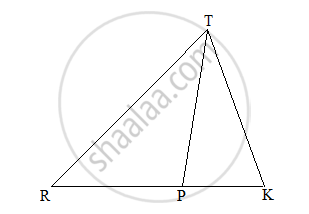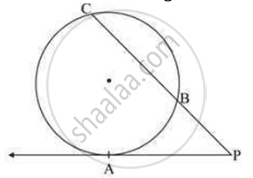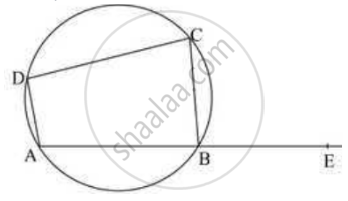Advertisement Remove all ads

# Geometry 2016-2017 SSC (Marathi Semi-English) 10th Standard [इयत्ता १० वी] Question Paper Solution

Advertisement Remove all ads
Geometry
Marks: 40Academic Year: 2016-2017
Date: July 2017

1 | Solve any five sub-questions:
1.i

In the following figure RP: PK= 3:2, then find the value of A(ΔTRP):A(ΔTPK).Concept: Properties of Ratios of Areas of Two Triangles
Chapter: [0.01] Similarity
1.ii

If two circles with radii 8 cm and 3 cm respectively touch externally, then find the distance between their centres.

Concept: Touching Circles
Chapter: [0.03] Circle
1.iii

If the angle θ= –60º, find the value of cosθ.

Concept: Trigonometric Ratios of Complementary Angles
Chapter: [0.06] Trigonometry
1.iv

Find the Slope of the line having inclination 45°.

Concept: Slope of a Line
Chapter: [0.04] Co-ordinate Geometry
1.v

Find the side of the square whose diagonal is 16sqrt(2) cm.

Concept: Right-angled Triangles and Pythagoras Property
Chapter: [0.02] Pythagoras Theorem
1.vi

Find the volume of a cube with side 6 cm.

Concept: Volume of a Combination of Solids
Chapter: [0.07] Mensuration
2 | Solve any four sub-questions:
2.i

Sides of a triangle are 7, 24 and 25. Determine whether the triangle is a right-angled triangle or not.

Concept: Similarity Triangle Theorem
Chapter: [0.01] Similarity
Advertisement Remove all ads
2.ii

The diameter of a sphere is 6 cm, Find the total surface area of the sphere. (π = 3.14)

Concept: Areas of Sector and Segment of a Circle
Chapter: [0.07] Mensuration
2.iii

Draw a tangent at any point ‘M’ on the circle of radius 3.3 cm and centre ‘O’.

Concept: Converse of Tangent Theorem
Chapter: [0.03] Circle
2.iv

If sinθ = 8/17, where θ is an acute angle, find the value of cos θ by using identities.

Concept: Application of Trigonometry
Chapter: [0.06] Trigonometry
2.v

Find the area of the sector of a circle of radius 8 cm and arc with length 15 cm.

Concept: Areas of Sector and Segment of a Circle
Chapter: [0.07] Mensuration
2.vi

In the given figure, a tangent segment PA touching a circle in A and secant PBC are shown, If AP = 15 cm BP = 10 cm, find the length of PC.Concept: Theorem of Angle Between Tangent and Secant
Chapter: [0.03] Circle
3 | Solve any the three sub-questions:
3.i

In ΔPQR, ∠P = 30°, ∠Q = 60°, ∠R = 90° and PQ = 12 cm, then find PR and QR.

Concept: Application of Trigonometry
Chapter: [0.06] Trigonometry
3.ii

ABCD is a cyclic quadrilateral. M (arc ABC) = 230°. Find ∠ABC, ∠CDA, and ∠CBE.Concept: Cyclic Quadrilateral
Chapter: [0.03] Circle
3.iii

Draw the circumcircle of ΔPMT in which PM = 5.6 cm, ∠P = 60°, ∠M = 70°.

Concept: Property of an Angle Bisector of a Triangle
Chapter: [0.01] Similarity
Advertisement Remove all ads
3.iv

Prove that:

sec2θ + cosec2θ = sec2θ x cosec2θ

Concept: Trigonometric Identities
Chapter: [0.06] Trigonometry
3.v

Find the equation of the line passing through the points (4,-5) and (-1,-2).

Concept: General Equation of a Line
Chapter: [0.04] Co-ordinate Geometry
4 | Solve any two sub-question:
4.i

Prove that the lengths of two tangent segments drawn to the circle from an external point are equal.

Concept: Number of Tangents from a Point on a Circle
Chapter: [0.03] Circle [0.03] Circle
4.ii

A person standing on the bank of river observes that the angle of elevation of the top of a tree standing on the opposite bank is 60°. When he moves 40 m away from the bank, he finds the angle of elevation to be 30°. Find the height of the tree and width of the river. (sqrt 3=1.73)

Concept: Heights and Distances
Chapter: [0.06] Trigonometry
4.iii

If P(-2,4), Q(4,8), R(10, 5) and S(4, 1) are the vertices of a quadrilateral, show that it is a parallelogram.

Concept: Converse: If a pair of opposite angles of a quadrilateral is supplementary, then the quadrilateral is cyclic.
Chapter: [0.03] Circle
5
5.i

Prove that “That ratio of areas of two similar triangles is equal to the square of the ratio of their corresponding sides.”

Concept: Basic Geometric Constructions
Chapter: [0.05] Geometric Constructions
5.ii

Draw a triangle ABC with side BC = 6cm, ∠B = 45° and ∠A = 100°, then construct a triangle PBQ whose sides are 7/4 times the corresponding sides of ΔABC.

Concept: Construction of Triangle If the Base, Angle Opposite to It and Either Median Altitude is Given
Chapter: [0.05] Geometric Constructions
Advertisement Remove all ads
5.iii

A tin maker converts a cubical metallic box into 10 cylindrical tins. The side of the cube is 50 cm and the radius of the cylinder is 7 cm. Find the height of each cylinder so made, if the wastage of 12% is incurred in the process (pi = 22/7)

Concept: Volume of a Combination of Solids
Chapter: [0.07] Mensuration
Advertisement Remove all ads

#### Request Question Paper

If you dont find a question paper, kindly write to us

View All Requests

#### Submit Question Paper

Help us maintain new question papers on Shaalaa.com, so we can continue to help students

only jpg, png and pdf files

## Maharashtra State Board previous year question papers 10th Standard [इयत्ता १० वी] Geometry with solutions 2016 - 2017

Maharashtra State Board 10th Standard [इयत्ता १० वी] Geometry question paper solution is key to score more marks in final exams. Students who have used our past year paper solution have significantly improved in speed and boosted their confidence to solve any question in the examination. Our Maharashtra State Board 10th Standard [इयत्ता १० वी] Geometry question paper 2017 serve as a catalyst to prepare for your Geometry board examination.
Previous year Question paper for Maharashtra State Board 10th Standard [इयत्ता १० वी] Geometry-2017 is solved by experts. Solved question papers gives you the chance to check yourself after your mock test.
By referring the question paper Solutions for Geometry, you can scale your preparation level and work on your weak areas. It will also help the candidates in developing the time-management skills. Practice makes perfect, and there is no better way to practice than to attempt previous year question paper solutions of Maharashtra State Board 10th Standard [इयत्ता १० वी].

How Maharashtra State Board 10th Standard [इयत्ता १० वी] Question Paper solutions Help Students ?
• Question paper solutions for Geometry will helps students to prepare for exam.
• Question paper with answer will boost students confidence in exam time and also give you an idea About the important questions and topics to be prepared for the board exam.
• For finding solution of question papers no need to refer so multiple sources like textbook or guides.
Advertisement Remove all ads
Share
Notifications

View all notifications

Forgot password?